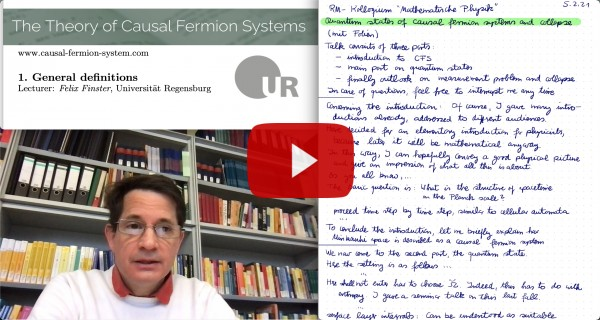# The Theory of Causal Fermion Systems

## Connection to Quantum Field TheorySo far, the connection between causal fermion systems and quantum field theory has been made on two levels:

## Description Starting from the Continuum Limit

In [qft13] the starting point are the effective field equations obtained in the continuum limit. In order to go beyond classical fields, the procedure is to complement the description by the so-called mechanism of microscopic mixing. This mechanism is based on the fact that causal fermion systems do allow for the description of systems which on the microscopic scale are composed of complicated mixtures of wave functions which may satisfy the Dirac equation for different bosonic potentials. Describing this mixing with random matrices, one gets an effective description of the interaction by a unitary time evolution on bosonic and fermionic Fock spaces.

While being a first step in the right direction, the procedure in [qft13] is not fully convincing for the following reasons:

• Working with random matrices reflects our lack of knowledge of the microscopic structure of spacetime. However, it is not so clear whether the microstructure really is “random” or whether it has more, yet unknown, structure. In other words, the use of random matrices still needs a convincing justification.
• The procedure requires assumptions (synchronization, instantaneous recombinations) which are ad-hoc and lack a fundamental explanation.
• Instead of starting from the effective field equations, one should better work on a more fundamental level with the Euler-Lagrange equations of the causal action principle.
• Similarly, the constructions are based on current conservation for the Dirac equation. In order to obtain a more fundamental description, one should work instead with the conservation laws for surface layer integrals.
• Finally, the procedure in [qft13] gives a connection to quantum field theory, but it does not seem to make it possible to go beyond quantum field theory in the sense of overcoming the shortcomings of present quantum field theory or of giving corrections to it.

## Description Starting from the Causal Action Principle

The above shortcomings were the motivation for getting a connection between causal fermion systems and quantum field theory starting directly from the causal action principle. The constructions make essential use of all the inherent structures of a causal fermion systems, in particular of the conservation laws for surface layer integrals and the related Fock space structures.

Restricting attention to bosonic interactions, these constructions are carried out in [FK18]. Indeed it is shown that the dynamics as described by the Euler-Lagrange equations can be reformulated in terms of a norm-preserving linear operator on ${\mathcal{F}}^* \otimes {\mathcal{F}}$. In the so-called holomorphic approximation, this operator is invariant on ${\mathcal{F}}$ and its dual, In this case, the time evolution can be described similar to quantum field theory by a unitary operator on the Fock space,

$U(t) \::\: {\mathcal{F}} \rightarrow {\mathcal{F}} \:.$

An important ingredient for obtaining a norm-preserving time evolution on Fock spaces is a surface layer integral which is nonlinear in the sense that it compares two measures $\rho$ and $\tilde{\rho}$ on $\F$ which do not need to be related to each other by jet transformations. The nonlinear surface layer integral takes the form

$\gamma^\Omega(\tilde{\rho}, \rho) = \int_{\tilde{\Omega}} d\tilde{\rho}(x) \int_{M \setminus \Omega} d\rho(y)\: \L(x,y) – \int_{\Omega} d\rho(x) \int_{\tilde{M} \setminus \tilde{\Omega}} d\tilde{\rho}(y)\: \L(x,y)$

The corresponding conservation law can be arranged by constructing a suitable mapping $F : M \rightarrow \tilde{M}$ and setting $\tilde{\Omega}=F(\Omega)$.

More recently, these constructions were extended in [FK21] in several ways: First, the fermions were included. Second, an abstract construction of a quantum state $\omega^t$ at time $t$ is given, being a positive linear mapping from the field algebra to the complex numbers. The definition of the state again involves the above nonlinear surface layer integral. Moreover, the unitary freedom in the identification of the Hilbert spaces of the vacuum spacetime $\rho$ and the interacting spacetime $\tilde{\rho}$ gives rise to an integral over a compact subgroup ${\mathcal{G}}$ of the unitary group $\rm{U}(\H)$. More precisely,

$\omega^t( \ldots ) = \frac{1}{Z^t} \int_{\mathscr{G}} (\cdots) \: e^{\beta \gamma^t(\tilde{\rho}, U \rho)}\: d\mu_{\mathscr{G}}(U) \:,$

where the insertions $(\cdots)$ on the right are complex-valued functions constructed from the field operators in the argument of $\omega^t$ on the left. Moreover, $\beta$ is a real parameter, and $Z^t$ is the partition function defined by

$Z^t := \int_{\mathscr{G}} e^{\beta \gamma^t(\tilde{\rho}, U \rho)}\: d\mu_{\mathscr{G}}(\rho) \:.$

In this description, Fock spaces arise only when constructing representations of the field algebra. Then the state is realized as the expectation of a density operator $\sigma^t$,

$\omega^t(A) = \text{tr}_{\mathcal{F}}(\sigma^t A) \:.$

The next step will be to analyze the time evolution of the state. In particular, one needs to construct a time evolution operator acting on the state,

${\mathfrak{L}}^t_{t_0} \::\: \omega^{t_0} \rightarrow \omega^t \:.$

Moreover, it needs to be analyzed whether this time evolution can be realized by a unitary operator $U^t_{t_0}$ on the Fock space,

$\sigma^t = U^t_{t_0}\, \sigma^{t_0}\, (U^t_{t_0})^{-1} \:.$

These problems are currently under investigation. In [FKR22], it is shown that the localized refined quantum state allows for the description of general entangled states. This analysis explains in detail how quantum entanglement is encoded in a causal fermion system.

There is a video of a seminar talk on the quantum state of a causal fermion system given in the Colloquium “Mathematical Physics Regensburg-Munich” in February 2021.